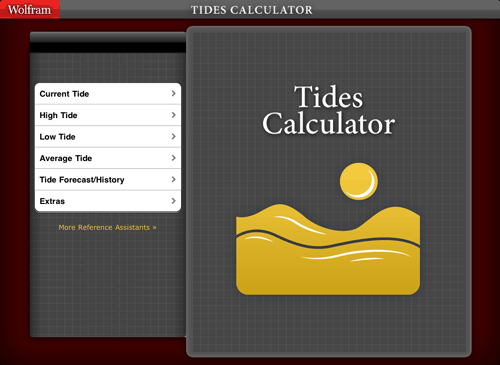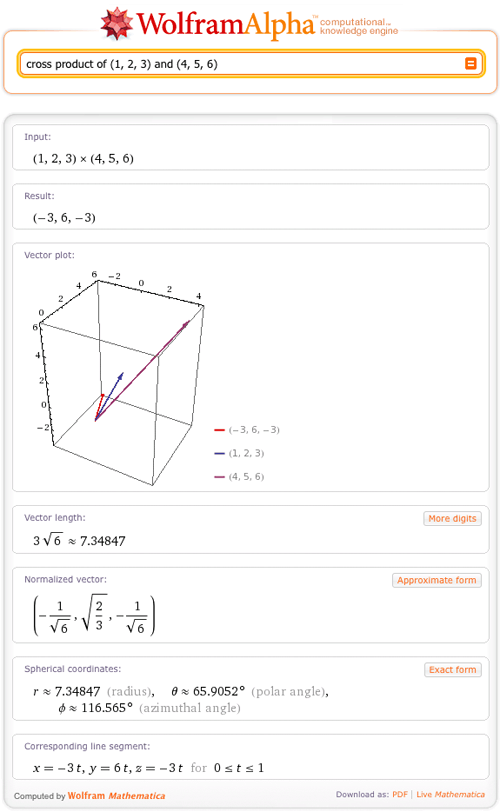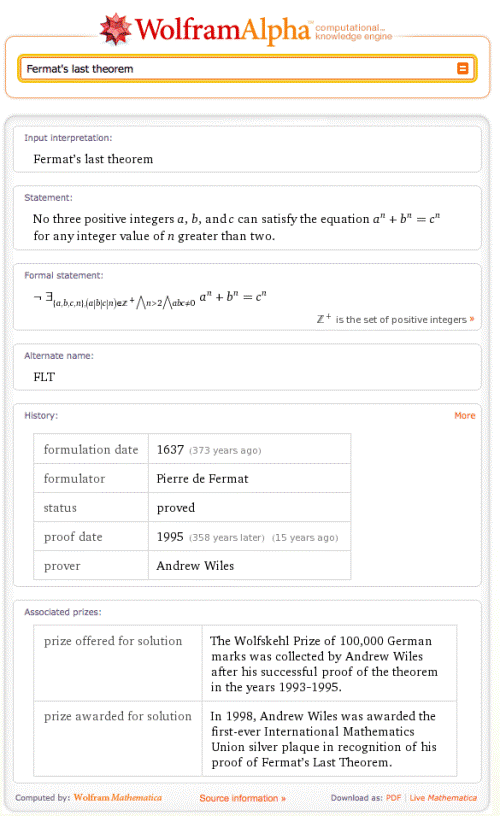TAG: Physics
June 7, 2011–

Today we are pleased to announce the Wolfram Tides Calculator and Wolfram Fractals Reference Apps for iPhone, iPod touch, and iPad. These are the first two in our series of reference apps that utilize Wolfram|Alpha technology to shed light on some fascinating subjects. Like our series of Wolfram Course Assistant Apps, the Wolfram Reference Apps are each designed with an optimized interface and specialized keyboards to enhance usability for mobile users.

The Wolfram Tides Calculator will become your go-to guide for tide information. Calculate the present tide or today’s high and low tides, do historical computations, or plan your vacation using the tide forecast. The app can automatically detect your current location or provide data from around the world.For example, with the Tides Calculator you can enter a future date and location to see the tide forecast for a specific date. Here is the prediction for tides in Miami on July 4:
More »

June 1, 2011–

What do you get when you cross a mountain climber with a mosquito? Nothing—you can’t cross a scalar with a vector!

But what do you get when you cross two vectors? Wolfram|Alpha can tell you. For example:And in fact, Wolfram|Alpha can give lots of information on vectors. A vector is commonly defined as a quantity with both magnitude and direction and is often represented as an arrow. The direction of the arrow matches the direction of the vector, while the length represents the magnitude of the vector. Wolfram|Alpha can now plot vectors with this arrow representation in 2D and 3D and return many other properties of the vector.
More »

May 4, 2010–

As we all know by now, Wolfram|Alpha is a computational knowledge engine. That means not only should it be able to do computations on a wide variety of topics, but also that it needs detailed knowledge of the names and salient properties of a wide variety of entities that are commonly encountered in human inquiry and discourse.

This is obvious in the case of classes of objects that fit neatly into curated data collections, such as mathematical surfaces (e.g., Möbius strip), countries of the world (e.g., New Zealand), chemicals (e.g., caffeine), and so forth.

What is perhaps slightly less obvious is just how much knowledge needs to be encoded to have a reasonable “understanding” of almost any named result in math and the sciences. For example, most people (including non-mathematicians) have heard of Fermat’s last theorem and therefore would rightly expect Wolfram|Alpha to be able to say something sensible about it.  And as one of my other hats involves writing the online encyclopedia of math known as MathWorld, which is hosted and sponsored by Wolfram Research, putting this information into Wolfram|Alpha naturally fell to me. So, for the past several months, I have been attempting to gradually build up Wolfram|Alpha’s knowledge base on named results in math and physics.

The screenshot below shows what Wolfram|Alpha now returns for Fermat’s last theorem:As you can see, Wolfram|Alpha begins by giving you the standard name for the result in question, followed by a clearly worded (or at least as clearly worded as could be managed in the marginal space available 😉 ) plain English statement of the result. Next, at least in cases where it is possible to do so, a mathematically precise “formal statement” of the result is given. This is followed by any common alternate names the result might have, a listing of historical information, and finally an enumeration of prizes associated with it (where relevant). More »